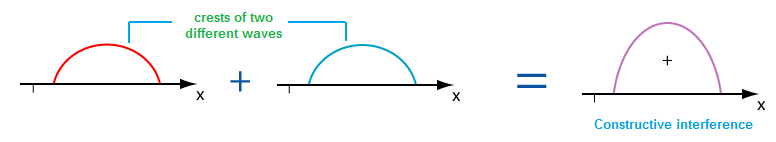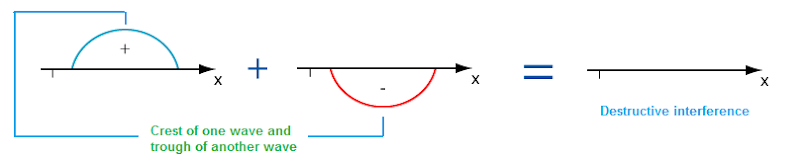# Types of Interference

When two waves meet each other, then they may either cancel each other or add up together. If the two waves are added together, it is called constructive interference, if they cancel each other, it is called destructive interference. In other words, during interference, dark and bright fringes (spots) are seen in the space which is called destructive interference and constructive interference.

Constructive interference:  The constructive interference is obtained at the point of the space when crest or trough of one wave falls upon the crest and trough of another wave at a point.Formation of constructive interference

The resultant amplitude at that point is sum of individual amplitude of the waves. So, energy becomes maximum at this point.

Path difference for constructive interference is given by:

Path difference = nλ

where n = 1, 2, 3…….etc.

Destructive interference: The destructive interference is obtained at the point of the space when crest of one wave falls upon the trough of another wave and vice versa.The resultant amplitude at that point given by the difference of amplitude of the two points. So, the energy becomes minimum at this point.

Path difference for destructive interference is given by:

Path difference = (n + 1/2)λ

Do you like this article ? If yes then like otherwise dislike :